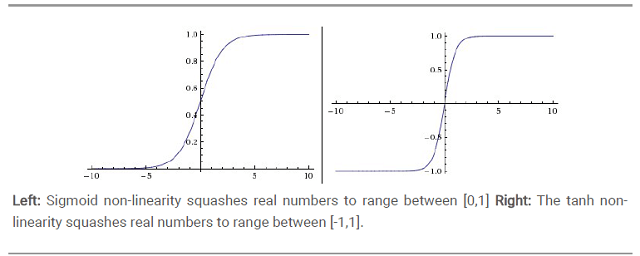# TensorFlow笔记5：神经网络中的激活函数(activation function)

## Jan 15,2018   3526 words   13 min

Tags: DeepLearning

#### 一、为什么要用激活函数

$y = w_{2-1}y_1+w_{2-2}y_2+w_{2-3}y_3$

$\left\{\begin{matrix} y_1 = w_{1-11}x_1+w_{1-12}x_2+b_{1-1} \\ y_2 = w_{1-21}x_1+w_{1-22}x_2+b_{1-2} \\ y_3 = w_{1-31}x_1+w_{1-32}x_2+b_{1-3} \end{matrix}\right.$

#### 二、激活函数是什么

• 非线性:当激活函数是线性的时候，一个两层的神经网络就可以逼近基本上所有的函数了。如果使用的是恒等激活函数，那么其实整个网络跟单层神经网络是等价的。
• 可微性:当优化方法是基于梯度的时候，这个性质是必须的。
• 单调性:当激活函数是单调的时候，单层网络能够保证是凸函数。
• f(x)≈x:当激活函数满足这个性质的时候，如果参数的初始化是random的很小的值，那么神经网络的训练将会很高效；如果不满足这个性质，那么就需要很用心的去设置初始值。
• 输出值范围:当激活函数输出值有限时，基于梯度的优化方法会更加稳定，因为特征的表示受有限权值的影响更显著；当激活函数的输出是无限时，模型的训练会更加高效，不过在这种情况小，一般需要更小的learning rate。

#### 三、常用的激活函数

##### 1.Sigmoid上图左边便是Sigmoid函数，表达式如下。

$f(x)=\frac{1}{1+e^{-x}}$

##### 2.tanh

tanh是上图中的右图，可以看出，tanh跟sigmoid还是很像的，实际上tanh是sigmoid的变形。

$tanh(x) = 2sigmoid(2x)-1$

##### 3.ReLU

$f(x) = max(0,x)$

$ReLU(x) = \left\{\begin{matrix} x\qquad x\geq 0\\ 0\qquad x< 0 \end{matrix}\right.$

##### 4.Leaky ReLUs

$ReLU(x) = \left\{\begin{matrix} x\qquad x\geq 0\\ \alpha x\qquad x< 0 \end{matrix}\right.$